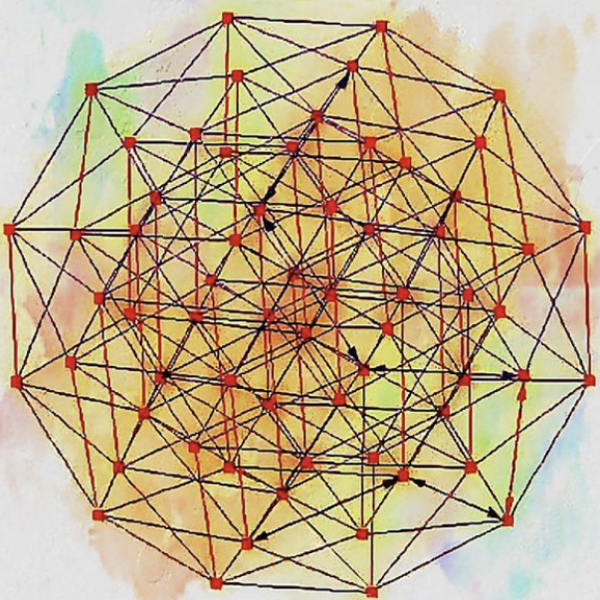# Point, line, square, cube, tesseract

Exploring the spectral properties of subgraphs of the hypercube and Hamming graphs for insights into coding theory and models of evolution.

The simplest model of dimension is the hypercube. When the coordinates are given by a binary string, the distance between two strings is the number of spelling mistakes between them. By considering strings made of up more than two symbols, and connecting strings that differ by just one spelling mistake, the hypercube can be generalized into Hamming graphs. In information theory, messages are sent as strings which are subject to errors in transmission. To correct these mistakes, variants of a string must code for the same message as the string itself. In this way error correction is deeply connected to partitioning the Hamming graph into compact clusters.

In this project, we investigate the spectral properties of subgraphs of the hypercube and Hamming graphs. By studying a diffusing population on these graphs, we develop an analogy between mutational robustness and the eigenvalues of subgraphs that code for the same phenotype.

The spectral theory of Hamming graphs has direct applications to the theory of neutral evolution, in which biological information is carried by strings of nucleotides or amino acids. It is also at the heart of Hamming and other error correcting codes, and Hamming graphs feature in association schemes in coding theory.## Papers in this project

•European Journal of Combinatorics

### Hypercube eigenvalues

Hamming balls, subgraphs of the hypercube, maximise the graph’s largest eigenvalue exactly when the dimension of the cube is large enough.

•Discrete Mathematics

### Eigenvalues of neutral networks

The principal eigenvalue of small neutral networks determines their robustness, and is bounded by the logarithm of the number of vertices.

•Forum of Mathematics, Sigma

•